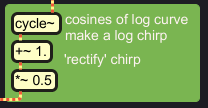# Fourier filter in Max/MSP and C

The Fourier filter principle as outlined on the previous page, was prototyped as a real time process in Max/MSP, using standard objects. The scheme for this is illustrated below. At the page bottom is a link where you can download the patchers.

Max/MSP has the convenient pfft~ object which can load any spectral subpatch featuring fftin~ and fftout~ objects. Window and overlap, FFT and IFFT are handled according to user parameter settings, performing the more efficient 'real FFT' routine if so desired. It is possibly the easiest doorway to real time spectral processing for a newbie like me.Below is the fourier filter subpatch which is loaded in pfft~. The pink noise test signal entering the subpatch via the fftin~ object is already windowed and transformed to real and imaginary spectrum coefficients. All the objects in the green blocks are there to compute a filter magnitude spectrum from the FFT bin index numbers. Only at the end, the input's spectrum coefficients reappear, when they are multiplied with the filter spectrum.Despite the blockwise lay-out, it may not be clear what is going on exactly, so I will comment the patch step by step.Start at the top left. A one-channel signal input is converted into a half-complex spectrum by MSP, with real part, imaginary part, and bin index number as the output streams. A logarithmic curve for each frame is computed with ln(index+1).The FFT size in this case is 2048 samples, which results in 1024 frequency bins. From this number, the spectrumsize, some parameters are computed in the top right block, in order to match the filter magnitude spectrum to the FFT size. 0.7/ln(spectrumsize) computes the minimum 'number' of filterbands that can be set, it is the floor norm. 3/ln(2) is the ceiling, independent from spectrumsize, and effectuating third-octave intervals. The floor is subtracted from the ceiling to compute a slope or deltafactor which is then multiplied by the user parameter. This result is applied as a factor on the initial logarithmic curve, and controls the number of filterbands.

Why will this 3/ln(2) effectuate third octave intervals, independent from spectrumsize? Actually this leads to ln(x)*3/ln(2) in the patch. That is equivalent to 2log(x)*3. While 2log(x) would do octave intervals, 2log(x)*3 makes a steeper curve, resulting in a faster sweep and logarithmically dividing octaves into thirds.Here, a user parameter of 10 bit value is entered to control a vertical shift of the logarithmic curve in the x-y plane. This will later result in an apparent horizontal shift of the sweep, though the sweep will change shape as well.Now at last the logarithmic curve is fed into a cosine lookup function, resulting in the sweep shape. The sweep is not rectified by an abs() function here, but scaled and vertically shifted to have positive values, from 0 till 1. This shape is slightly more advantageous for further manipulation.By raising the sweep to a positive power, it's lobes can be widened or narrowed, while keeping the y values in the 0 - 1 range. Negative powers are dangerous here, as these result in the muliplicative inverse of numbers smaller than 1, which can grow very large eventually. The user parameter is first reduced, then cubed, to optimise the parameter's resolution over it's range. Directly from the user parameter a magnitude compensation factor inbetween 1. and 2. is computed, and applied later on.

I found that the function raising the chirp to a power can easily generate so called denormal numbers: numbers smaller than can be represented by the single precision floating point type that is used for signals in Max/MSP. This will slow down signal processing, as denormals are handled as exceptions, and lead to thread blocks. Inserting a clip function here again, reduces the patcher's CPU load with some 20% of it's initial load.The sweep is subsequently multiplied by 1.5 and clipped at 1. The sinusoidal peaks become plateau's, creating filterbands with better definition.Finally, after magnitude compensation, the sweep is a filter magnitude spectrum, and as such multiplied with real and imaginary spectrum coefficients of the analysed signal. The filtered spectrum parts are resynthesized into a signal and that's it!I like to check the spectral process with a scope~ object. This is not the audio signal output, not even the spectrum of it! The scope is showing the filter characteristics, independent of the signal input. With a filter complicated like this, a visual check is almost indispensable.